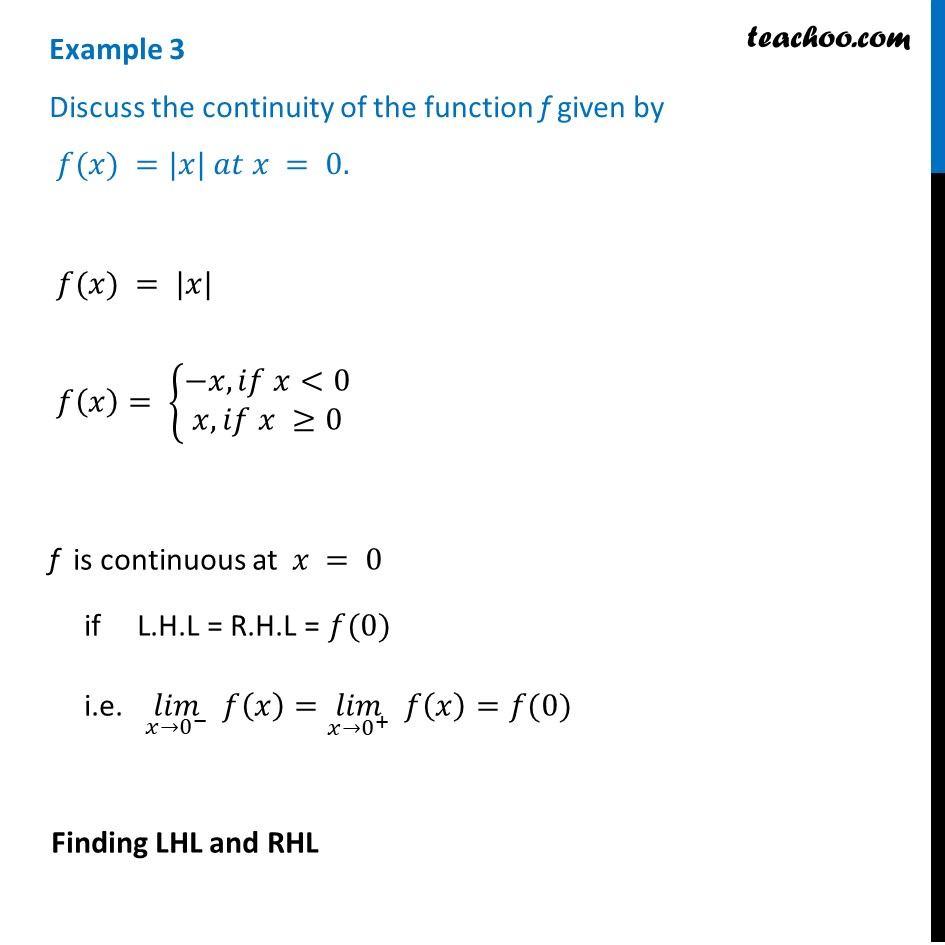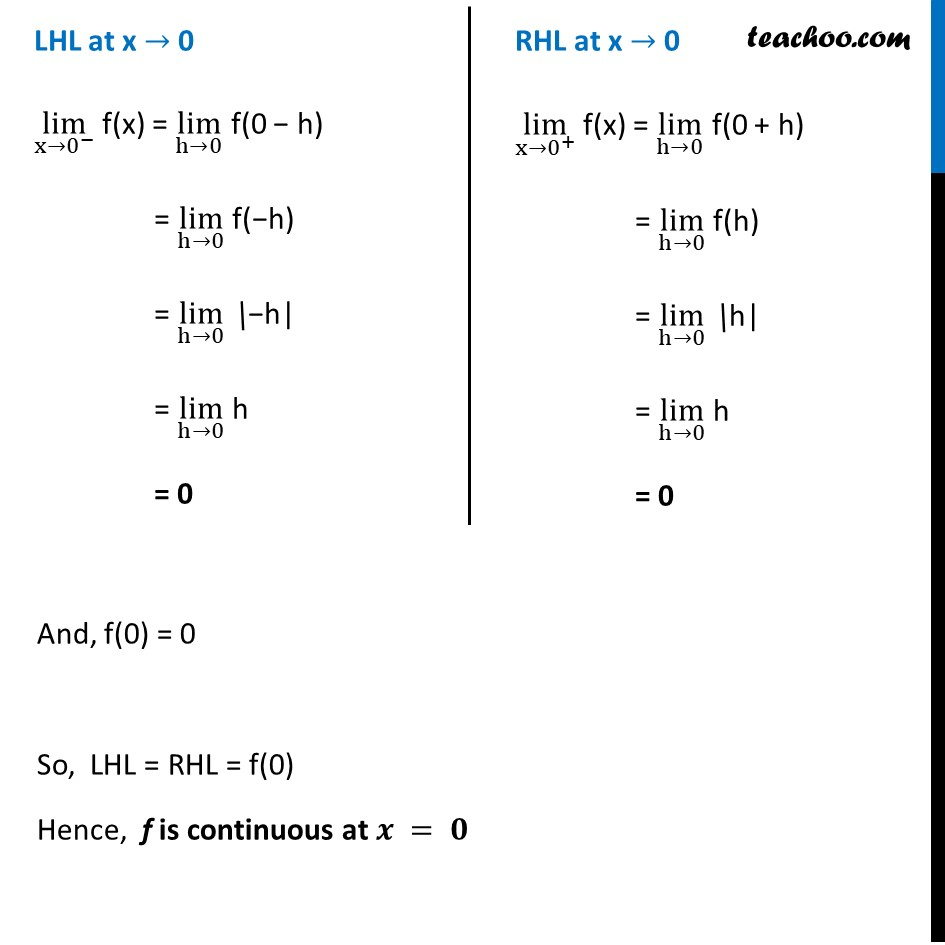Examples

Chapter 5 Class 12 Continuity and Differentiability
Serial order wiseLearn in your speed, with individual attention - Teachoo Maths 1-on-1 Class

### Transcript

Example 3 Discuss the continuity of the function f given by 𝑓(𝑥) =|𝑥| 𝑎𝑡 𝑥 = 0. 𝑓(𝑥) = |𝑥| 𝑓(𝑥)= {█(−𝑥, 𝑖𝑓 𝑥<0@𝑥, 𝑖𝑓 𝑥 ≥0)┤ f is continuous at 𝑥 = 0 if L.H.L = R.H.L = 𝑓(0) i.e. (𝑙𝑖𝑚)┬(𝑥→0^− ) 𝑓(𝑥)=(𝑙𝑖𝑚)┬(𝑥→0^+ ) 𝑓(𝑥)=𝑓(0) Finding LHL and RHL LHL at x → 0 lim┬(x→0^− ) f(x) = lim┬(h→0) f(0 − h) = lim┬(h→0) f(−h) = lim┬(h→0) \−h| = lim┬(h→0) h = 0 RHL at x → 0 lim┬(x→0^+ ) f(x) = lim┬(h→0) f(0 + h) = lim┬(h→0) f(h) = lim┬(h→0) \h| = lim┬(h→0) h = 0 And, f(0) = 0 So, LHL = RHL = f(0) Hence, f is continuous at 𝒙 = 𝟎